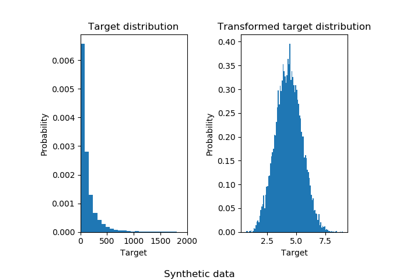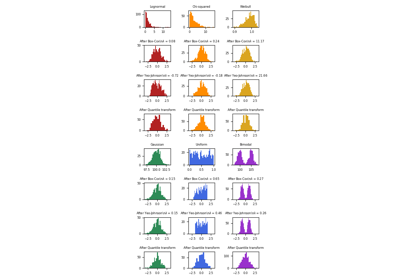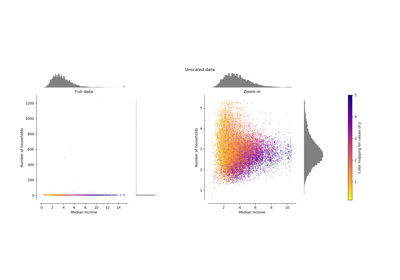# sklearn.preprocessing.QuantileTransformer¶

class sklearn.preprocessing.QuantileTransformer(n_quantiles=1000, output_distribution='uniform', ignore_implicit_zeros=False, subsample=100000, random_state=None, copy=True)[source]

Transform features using quantiles information.

This method transforms the features to follow a uniform or a normal distribution. Therefore, for a given feature, this transformation tends to spread out the most frequent values. It also reduces the impact of (marginal) outliers: this is therefore a robust preprocessing scheme.

The transformation is applied on each feature independently. The cumulative distribution function of a feature is used to project the original values. Features values of new/unseen data that fall below or above the fitted range will be mapped to the bounds of the output distribution. Note that this transform is non-linear. It may distort linear correlations between variables measured at the same scale but renders variables measured at different scales more directly comparable.

Read more in the User Guide.

Parameters: n_quantiles : int, optional (default=1000) Number of quantiles to be computed. It corresponds to the number of landmarks used to discretize the cumulative distribution function. output_distribution : str, optional (default=’uniform’) Marginal distribution for the transformed data. The choices are ‘uniform’ (default) or ‘normal’. ignore_implicit_zeros : bool, optional (default=False) Only applies to sparse matrices. If True, the sparse entries of the matrix are discarded to compute the quantile statistics. If False, these entries are treated as zeros. subsample : int, optional (default=1e5) Maximum number of samples used to estimate the quantiles for computational efficiency. Note that the subsampling procedure may differ for value-identical sparse and dense matrices. random_state : int, RandomState instance or None, optional (default=None) If int, random_state is the seed used by the random number generator; If RandomState instance, random_state is the random number generator; If None, the random number generator is the RandomState instance used by np.random. Note that this is used by subsampling and smoothing noise. copy : boolean, optional, (default=True) Set to False to perform inplace transformation and avoid a copy (if the input is already a numpy array). quantiles_ : ndarray, shape (n_quantiles, n_features) The values corresponding the quantiles of reference. references_ : ndarray, shape(n_quantiles, ) Quantiles of references.

quantile_transform
Equivalent function without the estimator API.
PowerTransformer
Perform mapping to a normal distribution using a power transform.
StandardScaler
Perform standardization that is faster, but less robust to outliers.
RobustScaler
Perform robust standardization that removes the influence of outliers but does not put outliers and inliers on the same scale.

Notes

NaNs are treated as missing values: disregarded in fit, and maintained in transform.

For a comparison of the different scalers, transformers, and normalizers, see examples/preprocessing/plot_all_scaling.py.

Examples

>>> import numpy as np
>>> from sklearn.preprocessing import QuantileTransformer
>>> rng = np.random.RandomState(0)
>>> X = np.sort(rng.normal(loc=0.5, scale=0.25, size=(25, 1)), axis=0)
>>> qt = QuantileTransformer(n_quantiles=10, random_state=0)
>>> qt.fit_transform(X)
array([...])


Methods

 fit(X[, y]) Compute the quantiles used for transforming. fit_transform(X[, y]) Fit to data, then transform it. get_params([deep]) Get parameters for this estimator. inverse_transform(X) Back-projection to the original space. set_params(**params) Set the parameters of this estimator. transform(X) Feature-wise transformation of the data.
__init__(n_quantiles=1000, output_distribution='uniform', ignore_implicit_zeros=False, subsample=100000, random_state=None, copy=True)[source]
fit(X, y=None)[source]

Compute the quantiles used for transforming.

Parameters: X : ndarray or sparse matrix, shape (n_samples, n_features) The data used to scale along the features axis. If a sparse matrix is provided, it will be converted into a sparse csc_matrix. Additionally, the sparse matrix needs to be nonnegative if ignore_implicit_zeros is False. self : object
fit_transform(X, y=None, **fit_params)[source]

Fit to data, then transform it.

Fits transformer to X and y with optional parameters fit_params and returns a transformed version of X.

Parameters: X : numpy array of shape [n_samples, n_features] Training set. y : numpy array of shape [n_samples] Target values. X_new : numpy array of shape [n_samples, n_features_new] Transformed array.
get_params(deep=True)[source]

Get parameters for this estimator.

Parameters: deep : boolean, optional If True, will return the parameters for this estimator and contained subobjects that are estimators. params : mapping of string to any Parameter names mapped to their values.
inverse_transform(X)[source]

Back-projection to the original space.

Parameters: X : ndarray or sparse matrix, shape (n_samples, n_features) The data used to scale along the features axis. If a sparse matrix is provided, it will be converted into a sparse csc_matrix. Additionally, the sparse matrix needs to be nonnegative if ignore_implicit_zeros is False. Xt : ndarray or sparse matrix, shape (n_samples, n_features) The projected data.
set_params(**params)[source]

Set the parameters of this estimator.

The method works on simple estimators as well as on nested objects (such as pipelines). The latter have parameters of the form <component>__<parameter> so that it’s possible to update each component of a nested object.

Returns: self
transform(X)[source]

Feature-wise transformation of the data.

Parameters: X : ndarray or sparse matrix, shape (n_samples, n_features) The data used to scale along the features axis. If a sparse matrix is provided, it will be converted into a sparse csc_matrix. Additionally, the sparse matrix needs to be nonnegative if ignore_implicit_zeros is False. Xt : ndarray or sparse matrix, shape (n_samples, n_features) The projected data.

## Examples using sklearn.preprocessing.QuantileTransformer¶Effect of transforming the targets in regression modelMap data to a normal distributionCompare the effect of different scalers on data with outliers